# Plotting in Sherpa Using Common Options## Overview

#### Synopsis:

This thread demonstrates the use of the plot_data and plot_fit commands to address the basic plotting needs of the typical Sherpa user.

Last Update: 7 Dec 2022 - updated for CIAO 4.15, typos fixed.

## Introduction

Sherpa uses the Matplotlib plotting package for 1-D (and some 2-D) visualization. This thread presents two examples of creating default Sherpa plots with commonly used Sherpa plotting commands, and modifying the plots using Matplotlib commands from the Sherpa prompt.No GUI

Prior to CIAO 4.12, ChIPS was used to create Sherpa plots, and it had a GUI with extensive capabilities for editing and adding data to plots. The GUI support provided by Matplotlib depends on the chosen "backend", but is limited in comparison to ChIPS.

## Getting Started

The sample data files used in this thread are available in sherpa.tar.gz, as explained in the Sherpa Getting Started thread, in the setplot_manual sub-directory directory:

```case1_1.pha
core1.arf
core1.rmf
```

In the first example, we create X-ray spectral plots, altering plotting preferences to create custom labels, colors, and axes ranges. In the second example, we use the same X-ray spectral profile, but re-plot it as a line plot instead of a scatter plot.

## Plotting X-ray Spectra

We begin by loading a PHA data set and its associated instrument response using load_pha, and filter the data to include only the range between 0.3-7.0 keV with notice_id. Then plotting the data is simply done with plot_data (Figure 1):

```sherpa> load_pha("case1_1.pha")
sherpa> notice_id(1, 0.3, 7.)
dataset 1: 0.00146:14.9504 -> 0.292:7.008 Energy (keV)

sherpa> plot_data()
```

### Figure 1: Screenshot of PHA Dataset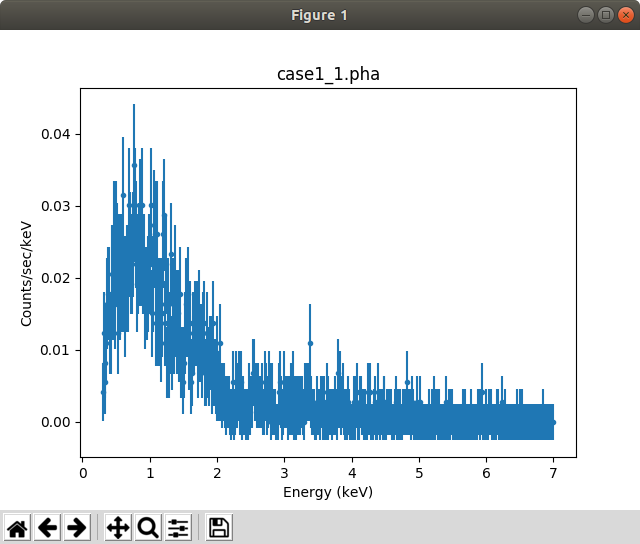### Figure 1: Screenshot of PHA Dataset

Plot of count-rate spectrum with the default plotting parameters.

A "hardcopy" version of the plot can be generated via the "save figure" button in the window or with the plt.savefig command (Figure 2):

```sherpa> plt.savefig('pha_data.png')
```

### Figure 2: Plot of PHA Dataset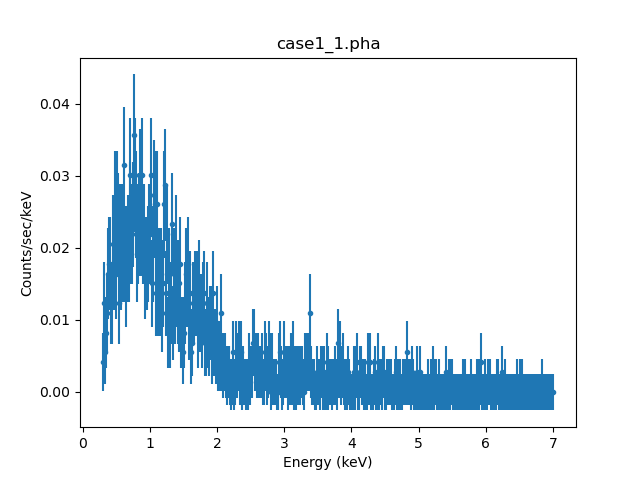### Figure 2: Plot of PHA Dataset

The PNG version of Figure 1 created with plt.savefig.

As we can see, Sherpa plots data in linear-scale by default, but sometimes, it is more useful to plot a logarithmic-scale. To switch to log-scale, we can use Matplotlib commands to change the existing plot; for instance

```sherpa> plt.xscale('log')
sherpa> plt.yscale('log')
sherpa> plt.ylim(0.001, 0.1)
(0.001, 0.1)
```

You can also set the xlog or ylog plot options when you call one of the plot functions (Figure 3):

```sherpa> plot_data(xlog=True, ylog=True)
sherpa> plt.ylim(0.001, 0.1)
(0.001, 0.1)
```

### Figure 3: Plot of PHA Dataset on Log-Scale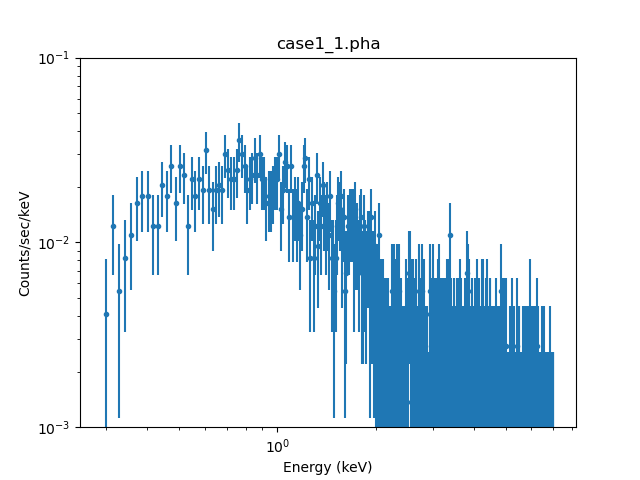### Figure 3: Plot of PHA Dataset on Log-Scale

Plot of count-rate spectrum on logarithmic-scale with the y-axis range set to 0.001-0.1 cts/sec/keV.

There are a number of ways to change the scale of plots, including:

When the plot is created

Set the xlog and ylog attributes of the plot call, which will over-ride any plot preference. This is shown above.

After the plot has been created

This is shown above with the plt.xscale() and plt.yscale() commands.

Changing the plot preferences

There are several ways to change the plot preferences:

1. The easiest is with the commands: set_xlog, set_ylog, set_xlinear, and set_ylinear. These can be used to change all plots, or a single type. For instance, we can set all plots to use a linear y-axis apart from residual-style plots (this does not include "ratio" or "delchi" style plots):

```sherpa> set_ylog()
sherpa> set_ylinear('resid')
```
2. The plot preferences can be changed directly (this is what the set_* commands do). The preferences can be retrieved with get_data_plot_prefs/get_model_plot_prefs, and changing an element of the returned dictionay will change the preferences. For example, to change data plots to always be displayed with a logarithmic scale for both axes you would say:

```sherpa> p = get_data_plot_prefs()
sherpa> p["xlog"] = True
sherpa> p["ylog"] = True
```

The default labelling for tickmarks with a logarithmic scale does not work well for Chandra-style data, but we can adjust this if required using the matplotlib ticker API. An example of this is shown below. First we create the plot (matching Figure 3):

```sherpa> plot_data(xlog=True, ylog=True)
sherpa> plt.ylim(0.001, 0.1)
(0.001, 0.1)
```

The tick formatting is controlled with (in this case) a simple string formatter:

```sherpa> import matplotlib.ticker as mtick
sherpa> tformatter = mtick.FormatStrFormatter("%g")
```

We can apply this new formatter using the gca function to obtain the current axes. This can then be used to change the formatting of both axes (Figure 4):

```sherpa> ax = plt.gca()
sherpa> ax.xaxis.set_major_formatter(tformatter)
sherpa> ax.yaxis.set_major_formatter(tformatter)
```

### Figure 4: Adjusting the tick labels for a logarithmic scale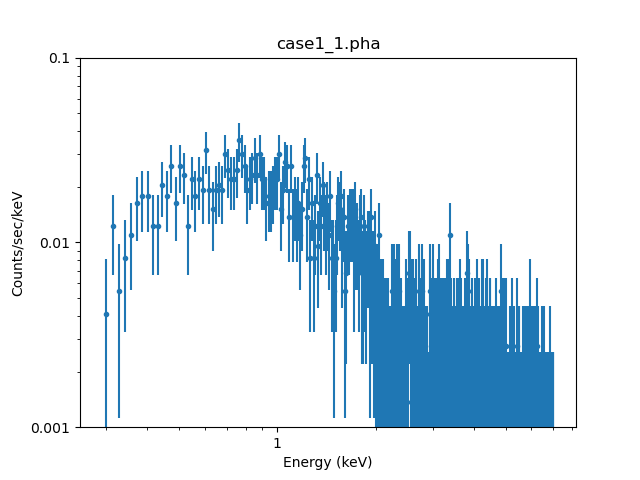### Grouping Data

In order to use Gaussian statistics to fit a model to a data set, it is often necessary to "group" the data—i.e., combine channels until you have enough counts—before use. It is possible to set and change the grouping of a file after it has been read into Sherpa by using the group commands: set_grouping, group, group_counts, group_snr, group_adapt, group_adapt_snr, group_bins, and group_width. (See the Sherpa thread Changing the grouping scheme of a data set within Sherpa for details.)

We use the group_counts function in this example to force each bin to have a minimum number of counts and apply the grouping after the filter, so we can use the tabStops argument to restrict the grouping to just the noticed data range.

```sherpa> notice_id(1, 0.3, 7.)
dataset 1: 0.292:7.008 Energy (keV) (unchanged)

```

The resulting plot is shown in Figure 5.

```sherpa> plot_data(xlog=True, ylog=True)
```

### Figure 5: Plot of Grouped PHA Dataset on Log-Scale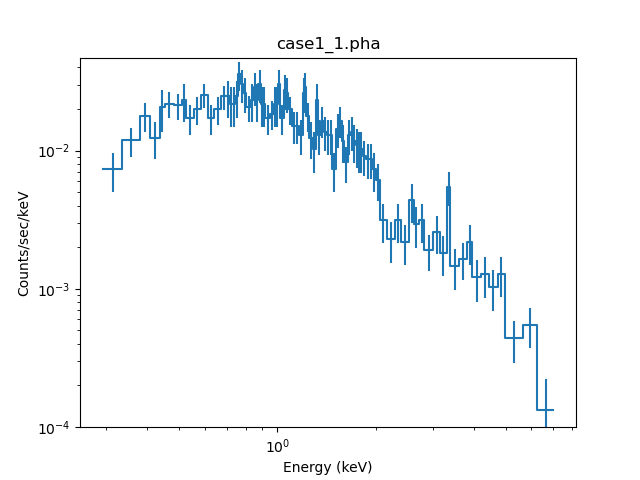### Figure 5: Plot of Grouped PHA Dataset on Log-Scale

Plot of the grouped, filtered count-rate spectrum on logarithmic-scale.

Notice that the noise/error-bars have been reduced by the grouping.Grouping and Filters

Note that after using a Sherpa group function, any notice or ignore filter previously applied will be reset if the data has been pre-grouped, e.g. with dmgroup, and must be re-applied, as the group functions restore use of the full range of the data in the analysis; however, if the data file is ungrouped, any applied notice/ignore filters will be maintained after grouping.

### Fitting a Model to Data

Next we will fit a simple, 1-D absorbed power-law model to the data and plot the fit using plot_fit (Figure 6). Note that by default, plot_fit creates a plot with data points marked by circles and associated error bars, and the model over-plotted as a solid orange line.

```sherpa> set_source(xsphabs.abs1 * powlaw1d.p1)
sherpa> fit()
Dataset               = 1
Method                = levmar
Statistic             = chi2gehrels
Initial fit statistic = 7.30455e+11
Final fit statistic   = 59.8111 at function evaluation 81
Data points           = 88
Degrees of freedom    = 85
Probability [Q-value] = 0.982656
Reduced statistic     = 0.70366
Change in statistic   = 7.30455e+11
abs1.nH        0.0343182    +/- 0.014151
p1.gamma       1.75149      +/- 0.0824047
p1.ampl        4.01875e-05  +/- 2.59962e-06

sherpa> plot_fit(xlog=True, ylog=True)
```

### Figure 6: Plot of Grouped PHA Dataset and Fitted Model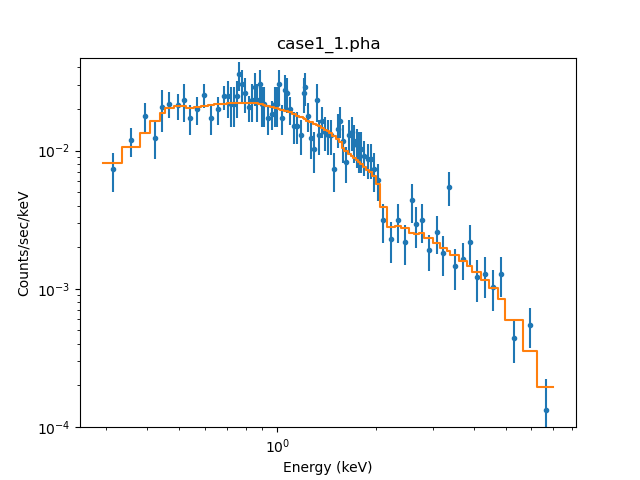### Figure 6: Plot of Grouped PHA Dataset and Fitted Model

The grouped, filtered count-rate spectrum on logarithmic-scale, with an absorbed power-law model fitted to the data (orange line).

The plot_model and plot_source commands are available for plotting the total convolved and unconvolved source model, respectively; and plot_model_component and plot_source_component plot one or a combination of individual model components contributing to a fit, where a multi-component source model is used (see the thread "Fitting a PHA Data Set with Multiple Responses" for a demonstration of this functionality).

The plot_source command supports the factor setting of the set_analysis command. This means that calling plot_source while the set_analysis 'factor' setting is 1 will plot E F(E) versus E in keV, or λ f(λ) versus λ in Angstroms, depending on which units are set for the spectral analysis. A set_analysis 'factor=2' setting will plot E2 F(E) versus E, or λ2 f(λ) versus λ.

```sherpa> plt.clf()
sherpa> plt.subplot(2, 1, 1)
sherpa> set_analysis("energy", factor=1)
sherpa> plot_source(xlog=True, ylog=True, clearwindow=False)

sherpa> plt.subplot(2, 1, 2)
sherpa> set_analysis("wavelength", factor=2)
sherpa> plot_source(xlog=True, ylog=True, clearwindow=False)

```

### Figure 7: Changing the axis units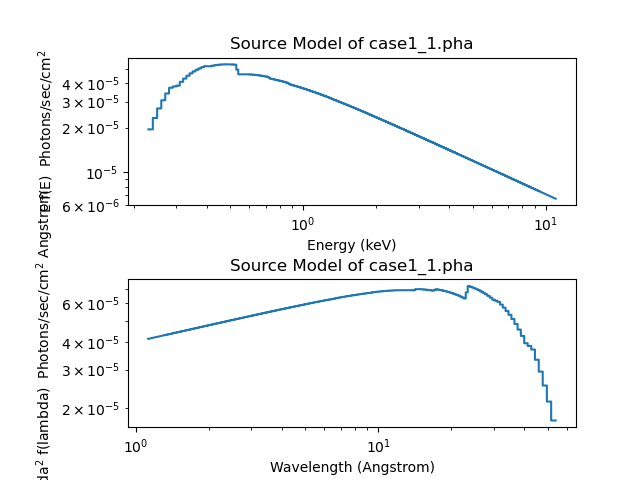### Figure 7: Changing the axis units

In the top plot the unconvolved source model for data set 1 is plotted in units of photons sec-1 cm-2 versus keV. In the bottom plot, the units are be Angstroms photons sec-1 cm-2 versus Angstroms.

Note the use of Matplotlib commands to create the two plots, and setting clearwindow=False in the plot_source calls to make sure the newly-created plot areas were not automatically erased.

Let's return to the default setting before going any further (and ensure we use logarithmic scales for the plot axes):

```sherpa> set_analysis('energy', factor=0)
sherpa> set_xlog()
sherpa> set_ylog()
```

### Creating Multi-Plots

We will now create a multi-plot with the plot_fit_delchi function, which plots the fitted spectrum and the δχ residuals of the fit.

```sherpa> plot_fit_delchi()
```

### Figure 8: Model Fit and δχ Residuals Multi-Plot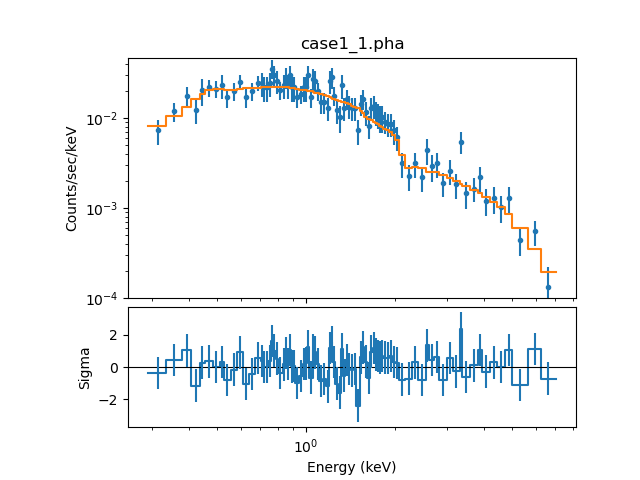### Figure 8: Model Fit and δχ Residuals Multi-Plot

The upper plot shows the data (blue points) with fitted model (orange), and the δχ residuals plot is shown on the bottom.

Prior to CIAO 4.13 the y-axis of the residual plot would have been also displayed with a logarithmic scale, which could have been "fixed" with a call to

```plt.yscale('linear')
```

but this is no-longer needed (the residual-style plots always use a linear scale for the y-axis).

The plot can be "inspected" and modified, using a variety of Matplotlib commands. The plt.gcf and plt.gca commands return objects that represent the current figure and axis-pair (respectively). The axes field of the figure lists all axis-pairs shown on the figure—in this case there are two of them (top plot and bottom plot):

```sherpa> fig = plt.gcf()
sherpa> ax1, ax2 = fig.axes
sherpa> print(ax1.get_ylabel())
Counts/sec/keV
sherpa> print(ax2.get_ylabel())
Sigma
```

By default the second (lower) plot is the "active" axis. That is shown by:

```sherpa> print(plt.gca().get_ylabel())
Sigma
```

The order of the plots can be shown by using the set_facecolor method on each axis, as shown in Figure 9:

```sherpa> ax1.set_facecolor('lightgray')
sherpa> ax2.set_facecolor('lightgreen')
```

### Figure 9: Changing the plots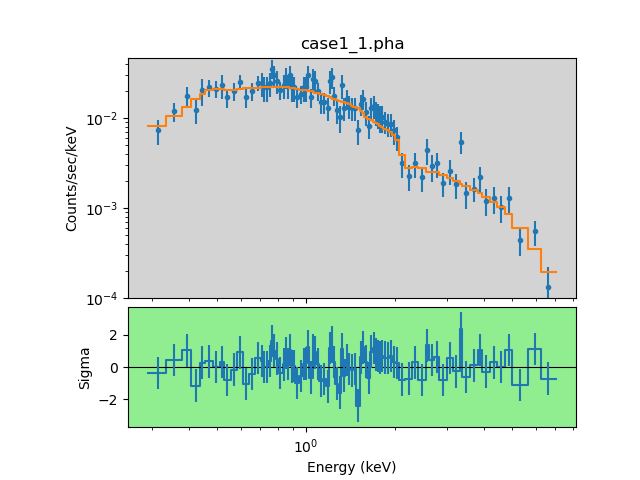### Figure 9: Changing the plots

Many things can be changed—e.g. the axis fonts, sizes, point styles and colors—but here we will concentrate on adding a label to the upper plot (Figure 10):

```sherpa> plt.sca(ax1)
sherpa> lbl = plt.text(0.3, 1e-3, 'Chandra', fontsize=14)
sherpa> lbl.set_position((1, 1e-3))
sherpa> lbl.set_horizontalalignment('center')
```

### Figure 10: Adding a label to a plot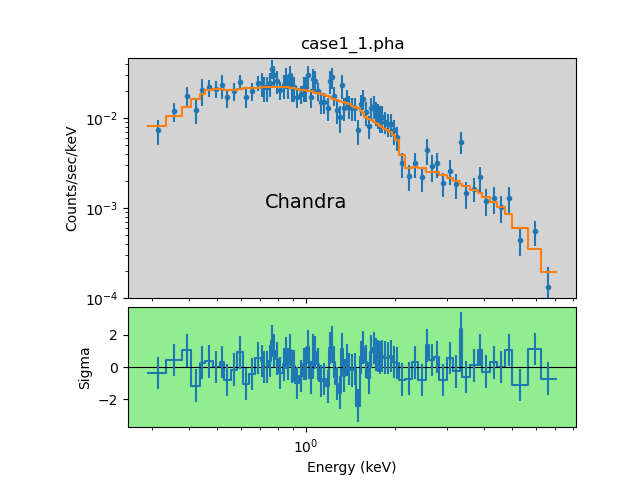### Figure 10: Adding a label to a plot

As we stored the object returned by plt.text we could use it to change the appearance of the label, in this case it's position. Tab-completion in an interactive environment like Sherpa is very helpful in situations like this (saving you from having to type out all of set_horizontalalignment!).

### Creating Plots of Multiple Datasets

As an aside, the Sherpa FAQ includes guides to creating many different types of plots, including those that display multiple datasets. The two following linked examples may be of interest to users:

## Changing a Scatter Plot to a Line Plot

We continue on with an example of how to modify the current scatter plot into a line plot.

First we over-ride the yerrorbars and xerrorbars preferences so that error bars are not shown (Figure 11):

```sherpa> plot_fit_delchi(yerrorbars=False, xerrorbars=False)
```

### Figure 11: Removing Error Bars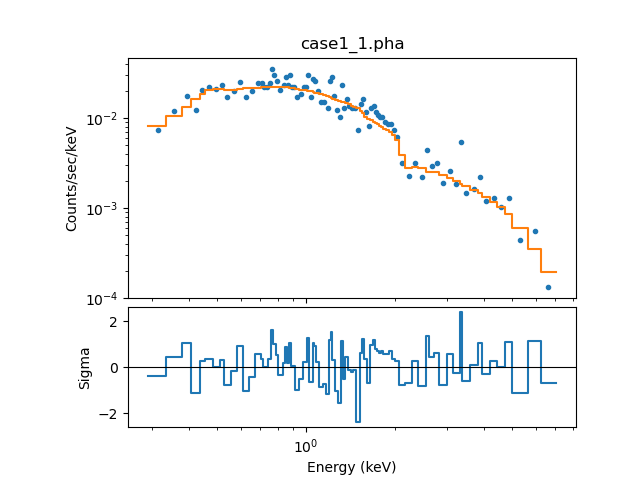### Figure 11: Removing Error Bars

Next, we modify the model fit plot by converting the data from symbols to a solid line (Figure 12):

```sherpa> ax1, ax2 = plt.gcf().axes
sherpa> print(ax1.lines)
<Axes.ArtistList of 4 lines>

sherpa> ax1.lines.set_linestyle('-')
sherpa> ax1.lines.set_marker(None)
```

### Figure 12: Removing Error Bars for a Spectral Profile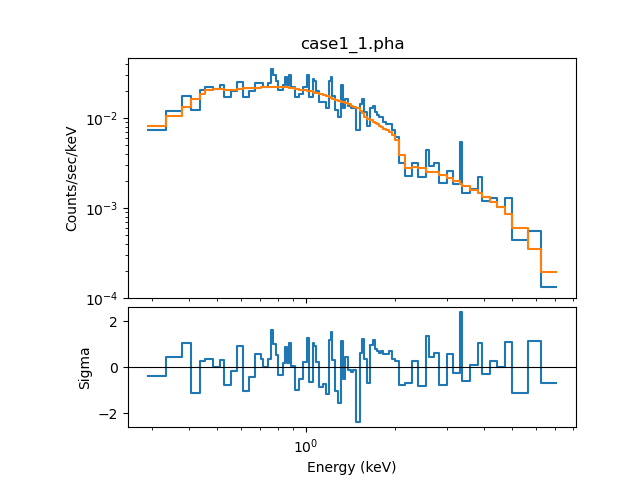### Figure 12: Removing Error Bars for a Spectral Profile

Changes made to the plot components—in this case the line—may not cause the display to update. The plot can be redrawn with a call to the redraw_in_frame method of the axis; in this case:

```sherpa> ax1.redraw_in_frame()
```

PHA-style plots were changed in CIAO 4.13 to use "histogram" style displays for data and plots. This has meant changes to the look (lines no-longer connect the centers of the bins) and the internal structure of the plot (e.g. ax1.lines now contains four elements, two for the data and two for the model, whereas in earlier CIAO versions there were only two elements).Changes to Sherpa plots

The plots created by Sherpa functions like plot_data and plot_fit_resid are not guaranteed to remain the same between CIAO versions, as they are updated to improve the display and take advantage of capabilities of the plotting library. This is of note for people who want to adjust the plots created by these commands, as it may depend on the version of CIAO in use as to what changes to make.

## Setting Default Plot Preferences

In order to change the default plot preferences for Sherpa plotting commands—e.g., to have plot_data create data plots with a logarithmic scaling by default, as opposed to linear—you should add the appropriate get_*_plot_prefs() command(s) to a Sherpa Python customization file, located in ~/.ipython-ciao/profile_sherpa/startup, as shown in the following example :

```unix% cat  ~/.ipython-ciao/profile_sherpa/startup/90-plot_customize.py
dplot = get_data_plot_prefs()
dplot['xlog'] = True
dplot['ylog'] = True
dplot['linestyle'] = '-'

mplot = get_model_plot_prefs()
mplot['xlog'] = True
mplot['ylog'] = True
```

These settings specify that the data pointed are connected with a solid-line when plotted, and both the x- and y-axes of data and model plots created in Sherpa should be drawn using a logarithmic scale, each time a function like plot_data or plot_fit is called. Note that adding the get_model_plot_prefs commands will not change the default axis scaling to logarithmic for model plots created with plot_model, it will remain linear. However, models plotted with plot_fit will be scaled logarithmically.

You must create a Python script that begins with a number, which is the order they will be called on, beginning with 00. Using get_data_plot_prefs() will show which plot options are configurable with this Python file.

## Writing Plots to File

Before exiting Sherpa, you may want to save your plot to file. In this example, we will create encapsulated Postscript, PDF, and PNG versions of the plot.

```sherpa> plt.savefig("line.eps", orientation="landscape")
sherpa> plt.savefig("line.pdf", orientation="landscape")
sherpa> plt.savefig("line.png")
```

## Scripting It

The file fit.py is a Python script which performs the primary commands used above; it can be executed by typing %run -i fit.py on the Sherpa command line.

The Sherpa script command may be used to save everything typed on the command line in a Sherpa session:

```sherpa> script(filename="sherpa.log", clobber=False)
```

(Note that restoring a Sherpa session from such a file could be problematic since it may include syntax errors, unwanted fitting trials, et cetera.)

The CXC is committed to helping Sherpa users transition to Matplotlib syntax from ChIPS. Please see the ChIPS to Matplotlib conversion guide for help, and contact the CXC Helpdesk if you still have problems.

## History

 04 Mar 2009 Created for CIAO 4.1 18 Mar 2009 New section on using ChIPS and Sherpa preferences 29 Apr 2009 new script command is available with CIAO 4.1.2 27 Jan 2010 Updated for CIAO 4.2: the print_window command no longer requires a 'format' specification; set_curve> and set_preferences now accept the 'err.*' and 'curve.err.*' shortform to display/hide error bars, respectively. The available Sherpa grouping commands are now listed in the "Grouping Data" section. 30 Jun 2010 added instructions for changing default plot preferences; udpates to plot_source in CIAO 4.2 Sherpa v2; S-Lang version of thread removed. 15 Dec 2010 updated for CIAO 4.3: new functions plot_model_component/plot_source_component, set_xlog/set_ylog, and set_xlinear/set_ylinear are available. 15 Dec 2011 reviewed for CIAO 4.4: the ChIPS GUI is available for modifying visualizations 27 Mar 2013 updated setting default plot preferences for CIAO 4.5, which uses a new iPython version. 11 Dec 2013 reviewed for CIAO 4.6: no changes 02 Apr 2014 added pointers to multi-plotting data. 27 Mar 2015 updated for CIAO 4.7, no content change. 10 Dec 2015 updated for CIAO 4.8, no content change. 09 Nov 2016 updated for CIAO 4.9, added more information on using LaTeX strings. 12 Dec 2019 Reworked for CIAO 4.12 as Matplotlib is now used instead of ChIPS and there have been minor improvements to the plot API in this release. 21 Dec 2020 Updated plots for CIAO 4.13, including removal of plt.yscale calls for residual plots (no-longer needed), and changes because of the plot changes for PHA datasets in CIAO 4.13. 04 Apr 2022 reviewed for CIAO 4.14, no content change. 07 Dec 2022 updated for CIAO 4.15, typos fixed.